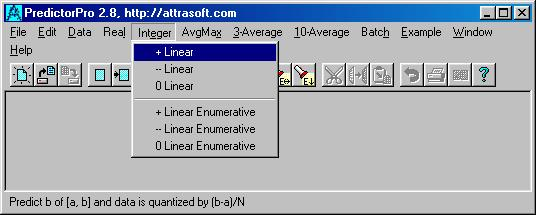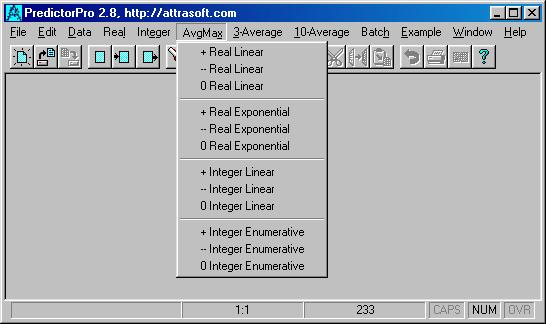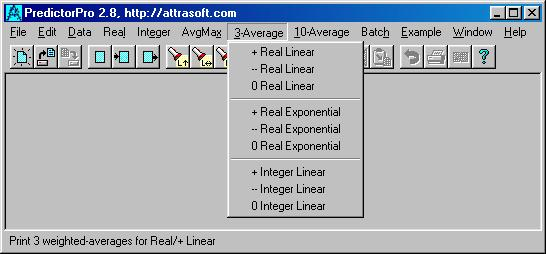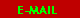# Attrasoft

PredictorPro for Windows

Search This Site

3.1 Step 1: Input File Format
3.1.1 Comment Section --- Part 1 of the Input File
3.1.2 Number of variables --- Part 2 of the Input File
3.1.3 Data Section --- Part 3 of the Input File
3.2 Step 2 and 3: Link and Run
3.3 Basic commends
3.4 Commands Lists
3.5 Output File
3.5.1 Relative Probability or Rating
3.5.2 Distribution
3.5.3 Error

3.  User's Guide

Chapter 2 is the quick version of the manual. Chapter 3 and 4 will present a detailed user's guide.

The operation has two phases:

Your Raw Data -- 90% of the work
• Set up a data set (a set of variables)
• Collect data
• Preprocessing if necessary

Using the PredictorPro -- 10% of the work

• Prepare input file
• Link this input file to the PredictorPro
• Run

In this chapter, we will discuss Phase 2: how to use the PredictorPro. Phase 2 has 3 steps:

1. From your raw data, prepare the input file according to the Attrasoft format.
2. Link the input file to the PredictorPro by clicking "File/Open" (first of 2 clicks).
3. Once the file is in the PredictorPro, click a command to get results (second of 2 clicks).

PredictorPro presents you with all possible predictions and how valuable the PredictorPro thinks each prediction is via a confidence number. This number is similar to the number in an Internet Search Engine: the higher that number, the more confidence the PredictorPro has in that prediction.

3.1 Step 1: Input File Format

The best way to learn the input file format is to look at the examples in the PredictorPro, for example, click "Example/Intel 2". The format is very easy to learn.

The input file must be prepared in the text file format. The PredictorPro has its own text editor. Or if you wish, you can use any word processor, like Microsoft Word, or WordPerfect; just make sure you save the data file in the text format.

The input file has three sections:

• Comment section;
• Number of variables section;
• Data section.

3.1.1 Comment Section --- Part 1 of the Input File

The comment section starts with a * and ends with another *. The purpose of this section is to document your data:

• Give a description of the data;
• Specify the data set used;
• Specify the beginning and the ending dates of the data
• . . .

Example:

*
Title:  Intel stock prediction
Data set : 5-month moving average of Intel
Date:   11/91 - 9/98
Purpose:            to predict the 5-month moving average, 10/98
*
Note: the PredictorPro will ignore this section. This section is for your remarks only.

3.1.2 Number of variables --- Part 2 of the Input File

This section has a single integer, which will tell the PredictorPro how many variables you will use.

Example:

In the Intel example in the last chapter, there was only one variable, therefore, the number of variables is 1. If you have 2 variables, then the number of variables is 2, ...

3.1.3 Data Section --- Part 3 of the Input File

This section contains the data.

Example  Considering the Intel example, which we showed you earlier. This example can be generated by clicking "Example/Intel 2". Note that it has three sections: comment, the number of variables, and the data:

*
Purpose: To predict next month Intel.
Data set: 5-month moving average of Intel, Nov-91 through Sep-98
Next 5-month moving average:
10/98           80.9378
*

1

6.7156
7.0282
7.0532
6.9814
6.8876
...

80.2004
78.8254
77.7754
76.4004
77.3878

3.2 Step 2 and 3: Link and Run

The operation of the Predictor is link and run. There are two methods of doing this. The first one deals with one or a few predictions. The second one deals with repeatedly making predictions from one data file.

Method 1:

• Link: use File/Open command to open a file. This will automatically link the file to the PredictorPro.
• Run: click a command under Real, Integer, Avg-Max, 3-Average or 10-Average menus.
Note:
You can check the linking by clicking the input-button (5th button).

Method 2:

• Click the input button (5th button);
• Cut and paste your data into this file, after clearing the old contents;
• Click the save-button (3rd button).

Run: click a command under Real, Integer, Avg-Max, 3-Average or 10-Average menus.

Note:

This method is a better one if you repeatedly make predictions from one data file. For example, your Excel data file has 10 columns and you want to make a prediction with each column. Method 1 requires you to create 10 new files, which makes data management difficult. Using Method 2, you can cut and paste one column into the PredictorPro, save the file and make a prediction; then repeat it for each column. In the end, you still have just one data file.

3.3 Basic commends

The basic commends are:

Real
+ Linear
-- Linear
0 Linear
+ Exponential
-- Exponential
0 Exponential

These commands are divided into two groups:

• Linear
• Exponential
The Linear mode deals with two situations:
• Data is in fixed intervals [a, b];
• Data grows linearly, 1, 2, 3, 4,   .

The Exponential mode deals with:

• Data grows exponentially, 1, 2, 4, 8,   .

Each group has three commands:

• --
• 0.
Here, "+" and "--" means the upper bound and the lower bound, respectively. Assume a prediction is

x = 5.6 + 0.3 - 0.2 ,

i.e. the result is likely to be 5.6, but could be in the range [5.4, 5.9]; then

'0' prediction ==> 5.6
'+' prediction ==> 5.9
'--' prediction ==> 5.4.

'+' and '--' predictions yield results more often than the '0' prediction, therefore, consider these two commands first. '0' prediction, on other hand, is more accurate than the other two, if it produces a prediction.

Example
Lottery numbers are good examples where the numbers are in a fixed interval. Intel stock is a typical example of the exponential mode.

3.4 Commands Lists

In this section, we will introduce the rest of the commands.

Real

+ Linear            basic command
-- Linear           basic command
0 Linear            basic command
+ Exponential   basic command
-- Exponential  basic command
0 Exponential   basic command

+ Linear Enumerative

Similar to + Linear command.

The difference between the linear command and the linear enumerative command is that the enumerative command might offer more possibilities than the liner mode.

The enumerative command looks at all possibilities where the linear command only looks at the important possibilities. However, the enumerative mode cannot examine more than 100,000 possibilities (for the 100,000-neuron version).

If your application requires a selection among more than 100,000 possibilities, you have to use the linear mode, or order a customized version. The 250K version, for example, will examine 250,000 possibilities.

-- Linear  Enumerative
Similar to -- Linear command.
0 Linear  Enumerative
Similar to 0 Linear command.Figure 7.  The "Integer" Commands

Integer

+ Linear
Similar to "Real/+ Linear" but uses integers.
-- Linear
Similar to "Real/+ Linear" but uses integers.
0 Linear
Similar to "Real/+ Linear" but uses integers.
+ Enumerative
Similar to "Real/+ Linear Enumerative" but uses integers.
-- Enumerative
Similar to "Real/-- Linear Enumerative" but uses integers.
0 Enumerative
Similar to "Real/0 Linear Enumerative" but uses integers.Figure 8.  The "Avg/Max" Commands

Avg/Max

+ Real Linear
Similar to "Real/+ Linear" but only prints the weighted average, most probable outcome, and the error.
-- Real Linear
Similar to "Real/-- Linear" but only prints the weighted average, most probable outcome, and the error.
0 Real Linear
Similar to "Real/0 Linear" but only prints the weighted average, most probable outcome, and the error.
+ Real Exponential
Similar to "Real/+ Exponential" but only prints the weighted average, most probable outcome, and the error.
-- Real Exponential
Similar to "Real/-- Exponential" but only prints the weighted average, most probable outcome, and the error.
0 Real Exponential
Similar to "Real/0 Exponential" but only prints the weighted average, most probable outcome, and the error.
+ Integer Linear
Similar to "Integer/+ Linear" but only prints the weighted average, most probable outcome, and the error.
-- Integer Linear
Similar to "Integer/-- Linear" but only prints the weighted average, most probable outcome, and the error.
0 Integer Linear
Similar to "Integer/0 Linear" but only prints the weighted average, most probable outcome, and the error.
+ Integer Enumerative
Similar to "Integer/+ Linear Enumerative" but only prints the weighted average, most probable outcome, and the error.
-- Integer Enumerative
Similar to "Integer/-- Linear Enumerative" but only prints the weighted average, most probable outcome, and the error.
0 Integer Enumerative
Similar to "Integer/0 Linear Enumerative" but only prints the weighted average, most probable outcome, and the error.Figure  9.  The "3-Average" Commands

3-Average and 10-Average

+ Real Linear
Similar to "Real/+ Linear". This command will make 3/10 predictions down the line; each time it will use the weighted-average(s) to calculate the next row of numbers.
-- Real Linear
Similar to "Real/-- Linear". This command will make 3/10 predictions down the line; each time it will use the weighted-average(s) to calculate the next row of numbers.
0 Real Linear
Similar to "Real/0 Linear". This command will make 3/10 predictions down the line; each time it will use the weighted-average(s) to calculate the next row of numbers.
+ Real Exponential
Similar to "Real/+ Exponential". This command will make 3/10 predictions down the line; each time it will use the weighted-average(s) to calculate the next row of numbers.
-- Real Exponential
Similar to "Real/-- Exponential". This command will make 3/10 predictions down the line; each time it will use the weighted-average(s) to calculate the next row of numbers.
0 Real Exponential
Similar to "Real/0 Exponential". This command will make 3/10 predictions down the line; each time it will use the weighted-average(s) to calculate the next row of numbers.
+ Integer Linear
Similar to "Integer/+ Linear". This command will make 3/10 predictions down the line; each time it will use the weighted-average(s) to calculate the next row of numbers.
-- Integer Linear
Similar to "Integer/-- Linear". This command will make 3/10 predictions down the line; each time it will use the weighted-average(s) to calculate the next row of numbers.
0 Integer Linear
Similar to "Integer/0 Linear". This command will make 3/10 predictions down the line; each time it will use the weighted-average(s) to calculate the next row of numbers.

3.5 Output File

The default output file name is example2b.txt. You can change the output file name by clicking: Data/Link, or its button on the toolbar, then type in your new data file name.

The "Real" and the "Integer" commands will have the following output format:

• a distribution, which is a list of possibilities and their probabilities;
• the weighted average and most probable outcome;
• errors.

The "Avg/Max" commands will have the following output format:

• the weighted average and most probable outcome;
• errors;
The "3-Average" and "10-average" commands will only produce 3 or 10 weighted-averages and their confidence.

Example   Intel 2.

1. Click "Example/Intel 2" to link the data to the PredictorPro. The input file will be opened at this point.

2. Click "Real/-- Exponential" and the output below will be displayed:

=================== Beginning =====================
Possibility              Confidence*Probability
81.2484       6400
77.8113       10344
76.0928       4235
84.6855       273
88.1226       1
86.404         33
79.5299       168
74.3742       8320
82.9669       261
---------------------------------------------------
Weighted Average
77.476
Highest Probability
77.8113  10344
Error of each number
0.859271
=================== End  ==========================

3.5.1 Relative Probability or Rating

Relative probability or rating is the ranking of the predicted numbers. This number is similar to an Internet Search Engine ranking number.

3.5.2 Distribution

PredictorPro presents you with rated possibilities: all possibilities and how valuable the PredictorPro thinks each one is via a relative probability. These ratings are relative probabilities. In the above example, ignoring results below 1000, the distribution is:

Possibility     Confidence*Probability
81.2484       6400
77.8113       10344
76.0928       4235
74.3742       8320
---------------------------------------------------

First of all, the predicted possibilities are

81.2484       ± 0.859271
77.8113       ± 0.859271
...

where ± 0.859271 is the given error in the last line of the output file.

Relative probabilities are proportional to probabilities. For example, let the relative probabilities be (3, 5), then they represent the probabilities (3/(3+5), 5/(3+5)) = (37.5%, 62,5%).

The highest relative probability is called the Confidence Number. This number is given after the weighted-average(s). The higher the number, the more confidence the PredictorPro has in that prediction. For each prediction, one Confidence Number is produced. In the above example, the confidence is 10,334.

Unfortunately, no quantitative description can be made for the Confidence Number. You can get a feel for this Confidence Number from experience. However, it is vitally important for you to acquire a feel for gauging this number. You should ignore the prediction results all together if the confidence number is low, as shown above.

3.5.3 Error

Of course, you want the error as low as possible. This is not always possible. The error is controlled by a user-defined variable, called Precision level. The higher Precision level is, the lower the error will be. We will discuss the Precision level in the next chapter.

Online Order/Online Fax order:
Attrasoft PredictorPro for Windows
Version 2.8 (\$494.99 + \$5 US Shipping and Handling)Mail Order: 499.99 (S&H included)

PredictorPro
Attrasoft, Attn.: Gina
P. O. Box 13051
Savannah, GA. 31406, USA
Send questions or comments to:webmaster@attrasoft.com